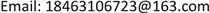1. 引言

2. 模型建立

2.1. 出行方式选择模型

p r s i j = e − γ ( u r s i j + υ j λ r s − θ i M j ) ∑ m ∈ M e − γ ( u r s i m + υ m λ r s − θ i M m ) ,   ∀ i , r , s , m (1)

2.2. 下层多方式网络均衡模型

min f z ( f , Q ) = ∑ l ∈ L ∫ 0 x l t l ( w ) d w + 1 γ ∑ r ∈ R ∑ s ∈ S ∑ m ∈ M ( q r s m ln q r s m − q r s m ) − ∑ r ∈ R ∑ s ∈ S ∑ m ∈ M ∑ i ∈ I θ i M m q r s i m (2)

∑ k ∈ K r s m f i k r s = q r s i m , ∀ r , s , i , m (3)

∑ s ∈ S ∑ m ∈ M q r s i m = O r i , ∀ r , i (4)

q r s a ≤ Q r s , ∀ r , s (5)

f i k r s ≥ 0 , k ∈ K r s m , ∀ m , r , s , i , k (6)

x l = ∑ i ∈ I ( ∑ r ∈ R ∑ s ∈ S ∑ k ∈ K r s a f i k r s δ l k r s + ∑ r ∈ R ∑ s ∈ S ∑ k ∈ K r p c f i k r p δ l k r p ) , l ∈ L a (7)

x l = ∑ i ∈ I ( ∑ r ∈ R ∑ s ∈ S ∑ k ∈ K r s b f i k r s δ l k r s + ∑ r ∈ R ∑ s ∈ S ∑ k ∈ K p ' s c f i k p ′ s δ l k p ′ s ) , l ∈ L b (8)

x l = ∑ r ∈ R ∑ s ∈ S ∑ m ∈ M ∑ k ∈ K r s m ( ∑ i ∈ I f i k r s ) ⋅ δ l k r s , ∀ l ∈ L (9)

2.3. 上层停车券分配模型

min h ( Q r s ) = ∑ l ∈ L t l ( x l ) ⋅ x l (10)

∑ r ∈ R Q r s ≤ Q s , ∀ s (11)

Q r s ≥ 0 , ∀ r , s (12)

Q r s 是上层规划的输入变量，为终点分配给起点r的停车券数量， x l 是下层规划问题的解，是关于 Q r s 的函数。约束(11)保证终点分配给所有起点的停车券总量应不超过其自身的停车容量( Q s )限制。

3. 求解算法

min 1 2 ‖ Q − Q ^ ‖ 2 (13)

1) 利用均匀分布进行随机扰动，产生一个新的试探点 p ^ 。若 p ^ 满足上层约束，则取 p = p ^ ，否则令 Q ^ = p ^ ，求解问题(13)得到最优解p。以p为上层决策变量取值，求解下层规划，得到最优解 f ˜ ，计算下层目标函数值 h ˜ 。

2) 若 h n − h ˜ > 0 ，则接受p，令， f n + 1 = f ˜ ， h n + 1 = h ˜ 。否则，以一定的概率决定是否接受新解：产生一个(0,1)上均匀分布的随机变量 σ ，计算在当前迭代点和温度 T k 下接受点p的概率

ρ = exp ( h n − h ˜ T k ) 。若 σ < ρ ，则令 Q n + 1 = p ， f n + 1 = f ˜ ， h n + 1 = h ˜ ；否则， Q n + 1 = Q n ， f n + 1 = f n ， h n + 1 = h n 。

3) 更新 n = n + 1 ，若 n < L N 时，继续下一次的扰动和接受过程，即执行1) 2)过程。否则，转到步骤3。

4. 算例分析

4.1. 参数设置

t l ( x l ) = t l 0 [ 1 + α ( x l C l ) β ] , ∀ l ∈ L (14)

(1,4)道路102400(6,5)道路71200
(4,7)道路132400(9,8)道路51200
(7,10)道路152400(5,15)换乘82000
(2,5)道路121200(1,11)地铁124000
(5,8)道路101200(11,12)地铁104000
(8,10)道路81200(12,13)地铁84000
(4,5)道路61200(13,16)地铁74000
(7,8)道路41200(2,14)地铁104000
(3,6)道路102400(14,15)地铁84000
(6,9)道路122400(15,16)地铁74000
(9,10)道路142400(16,10)地铁64000

γ 取值为1。

4.2. 算例结果和效率分析

Traffic equilibrium results with optimal parking permit distributio
OD对出行方式OD对出行量总计

(1,10)小汽车5880588

(2,10)小汽车000

(3,10)小汽车191215003412

5. 结论